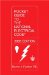# Example No. D6 Maximum Demand for Range Loads

Table 220.55, Column C, applies to ranges not over 12 kW. The application of Note 1 to ranges over 12 kW (and not over 27 kW) and Note 2 to ranges over 8 3/4 kW (and not over 27 kW) is illustrated in the following two examples:

A. Ranges all the same rating (See Table 220.55, Note 1)

Assume 24 ranges each rated 16 kW. From Table 220.55, Column C the maximum demand for 24 ranges of 12 kW rating is 39 kW.

16 kW exeeeds 12 kW by 4

5% x 4 = 20% (5% increase for each kW in excess of 12)

39 kW x 20% = 7.8 kW increase

39 kW + 7.8 kW = 46.8 kW (value to be used in selection of feeders)

B. Ranges of unequal rating (See Table 220.55, Note 2)

Assume:

5 ranges each rated 11 kW

2 ranges each rated 12 kW

20 ranges each rated 13.5 kW

3 ranges each rated 18 kW

 5 x 12 = 60 kW (Use 12 kW for range rated less than 12) 2 x 12 = 24 kW 20 x 13.5 = 270 kW 3 x 18 = 54 kW 30 ranges 408 kW total kW

408 kW ÷ 30 ranges = 13.6 kW (average to be used for calculation)

From Table 220.55, Column C, the demand for 30 ranges of 12 kW rating is 15 kW + (1 kW x 30 ranges) = 45 kW.

13.6 exceeds 12 by 1.6 (use 2 kW)

5% x 2 = 10% (5% increase for each kW in excess of 12 kW)

45 kW x 10% = 4.5 kW increase

45 kW + 4.5 kW = 49.5 kw: (value to be used in selection of feeders)Pocket Guide to the National Electrical Code(R), 2005 Edition (8th Edition)
ISBN: 0131480014
EAN: 2147483647
Year: 2004
Pages: 120